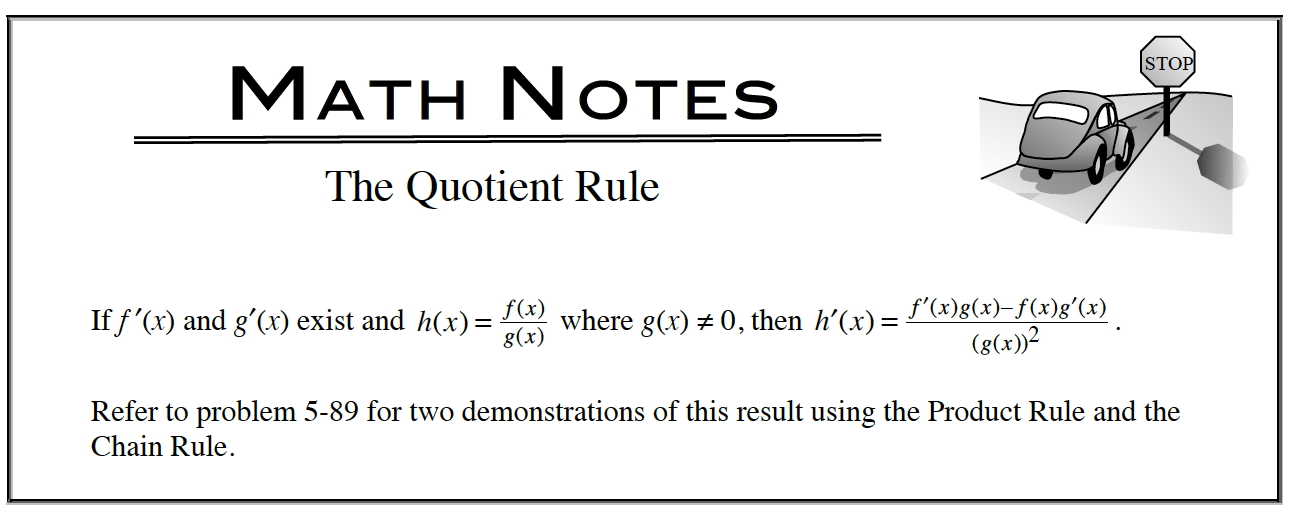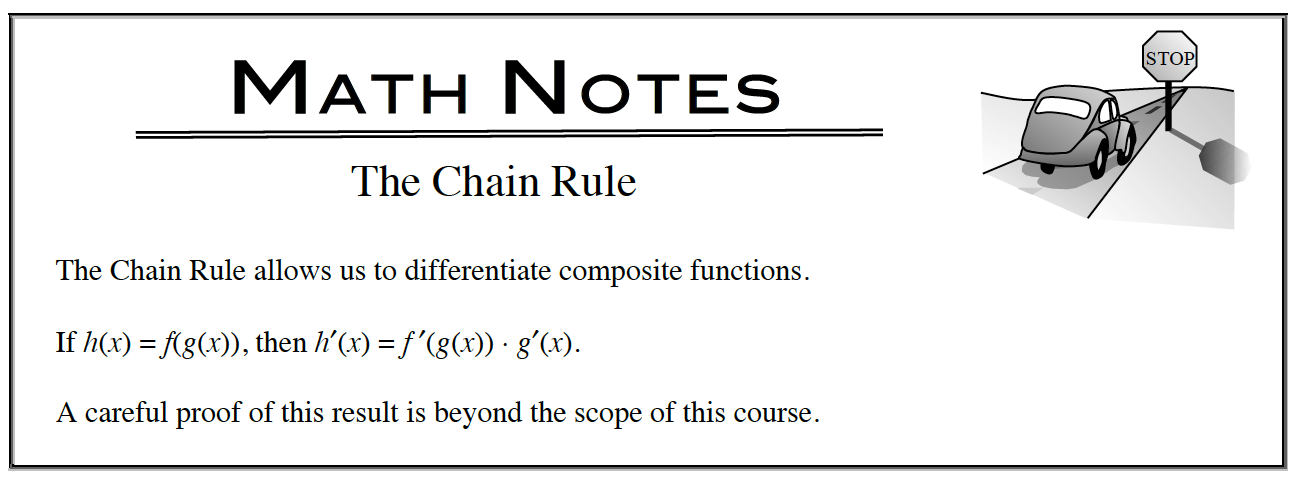### Home > APCALC > Chapter 5 > Lesson 5.2.5 > Problem5-106

5-106.

Use your derivative tools to write the second derivative, $\large\frac { d ^ { 2 } y } { d x ^ { 2 } }$, of each function below. Homework Help ✎

1. $y=\frac{\sin(x)}{x}$2. $y=\csc^2\left(x\right)-\cot^2\left(x\right)$3. $y=\sqrt{\frac{1}{x}}$

You could use the quotient rule AND the chain rule...Or you could rewrite y as with exponents and quickly use the Power Rule!

4. $y=|x-2|$

Rewrite y as a piecewise function. The derivative will be a piecewise function as well. So will the second derivative.